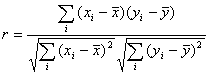# Correlation

Let's consider the following problem. A set of measurements of random variables X and Y was carried out. At that, X and Y were measured in pairs, and so, after each measurement we get two values - x and y. Having a sample of pairs (x, y), we are to decide whether these variables depend on each other or not.

Dependence between two variables can be functional (i.e. there is a strict functional relationship between X and Y). However, more often series of experimental data have another kind of dependence: statistical dependence. The difference between these two types of dependences is that functional dependence always defines strict relationships between variables, whereas statistical dependence implies that only distribution of Y depends upon the value of X.

One of the statistical dependence measures is the correlation coefficient. It indicates how one variable tends to increase on the condition that another variable is increasing too. Correlation coefficient belongs to the interval [-1, 1]. Zero value indicates the absence of such dependence (but not necessary the absence of dependence at all). If tendency is pronounced, the correlation coefficient is close to -1 or +1 (depending on sign). If the correlation coefficient equals -1 or +1, the variables have functional dependence. Intermediate values indicate that the tendency of increasing (decreasing) both values simultaneously exists, but is not pronounced.

# Pearson's correlation coefficient

There are several correlation coefficients. The most popular coefficient is Pearson's correlation coefficient. It characterizes the degree of linear dependence between variables. It is defined asThis correlation coefficient is calculated by PearsonCorrelation subroutine.

It should be taken into account that this coefficient is used to estimate a relation between two normal random variables. If the distribution is far from normal, the coefficient still characterizes the degree of dependence, but you can't apply significance tests to it. Pearson's correlation coefficient is not stable to outliers. If the random variable has outliers, you can draw the wrong conclusion about the presence of a correlation. Therefore, if the distribution is far from normal or the variable can have outlier values, it's better to use Spearman's rank correlation coefficient.

# Spearman's rank correlation coefficient

If we take variable ranks instead of their values and calculate Pearson's correlation coefficient for this sample, we get a non-parametric correlation coefficient which is called Spearmen's rank correlation coefficient. Unlike Pearson's correlation coefficient, it characterizes a degree of arbitrary non-linear dependence within the "if one variable increases, the second variable increases too" model. This correlation coefficient is calculated by SpearmanRankCorrelation subroutine.

It should be noted that Spearman's correlation coefficient could be used to estimate a relation between variables independently of their distribution. This quality is attained due to the fact that distribution-specific information is lost when the data are replaced by their ranks. This coefficient is also less sensitive to the outliers, which is important when working with experimental data.

ALGLIB Project offers you two editions of ALGLIB:

ALGLIB Free Edition:delivered for freeoffers full set of numerical functionalityextensive algorithmic optimizationsno low level optimizationsnon-commercial license

ALGLIB Commercial Edition:flexible pricingoffers full set of numerical functionalityextensive algorithmic optimizationshigh performance (SMP, SIMD)commercial license with support plan

## ALGLIB 3.15.0 for C++C++ library.
Delivered with sources.
Monolithic design.
Extreme portability.
Editions:   FREE   COMMERCIAL

## ALGLIB 3.15.0 for C#C# library with native kernels.
Delivered with sources.
VB.NET and IronPython wrappers.
Extreme portability.
Editions:   FREE   COMMERCIAL

## ALGLIB 3.15.0 for DelphiDelphi wrapper around C core.
Delivered as precompiled binary.
Compatible with FreePascal.
Editions:   FREE   COMMERCIAL

## ALGLIB 3.15.0 for CPythonCPython wrapper around C core.
Delivered as precompiled binary.
Editions:   FREE   COMMERCIAL